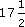Our everyday experience of percentages includes percentage increases (like VAT at%, or a service charge of 15%) and percentage decreases (such as a discount of 15%).

For example, £8 plus
Author(s): The Open University

## Activity 20

Convert each of the following to percentages. Round off the percentages to whole numbers.

• (a)

• (i) 0.8

• (ii) 0.
Author(s): The Open University

Fractions and decimals can also be converted to percentages, by multiplying by 100%.

So, for example, 0.17, 0.3 andcan be expressed as percentages as follows:

0.17 × 100% = 17%;

<
Author(s): The Open University

## Activity 17

Express each of the following percentages as fractions:

• (a) 40%

• (b) 8%

• (c) 70%

• (d)
Author(s): The Open University

Percentages are used, particularly in newspaper articles, to indicate fractions (as in ‘64% of the population voted’) or to indicate changes (as in ‘an increase of 4%’).

Percentages often indicate proportions. For example, labels in clothes indicate the various proportions of different yarns in the fabric. ‘Per cent’ means ‘per hundred’ and is denoted by the symbol %. 100% is the same as the whole, or one hundred per hundred.

Author(s): The Open University

## Activity 14

A piece of computer software is to be developed by a team of programmers. It is estimated that a team of four people would take a year. Which of the following times is the length of time taken by three program
Author(s): The Open University

In Section 2.2 you saw that direct proportion described relationships between two quantities, where as one increased, so did the other. Sometimes as one quantity increases the other decreases instead of increasing. This is called indirect proportion. Team tasks are often an example of this. The ti
Author(s): The Open University

## Activity 12

A recipe for four people calls for [Image_Link]https://www.open.edu/openlearn/ocw/pluginfile.php/104648/mod_oucontent/oucontent/1902/84dcd0a9/ac42
Author(s):
The Open University

In a recipe the quantity of each ingredient needed depends upon the number of portions. As the number of portions increases, the quantity required increases. The quantity per portion is the same. This is called direct proportion. The quantity is said to be directly proportional to the number of portions. If 2 potatoes are required for one portion, 4 will be required for two portions etc. A useful method for direct proportion problems is to find the quantity for one and multiply by the
Author(s): The Open University

## Activity 5

Convert each of the following fraction ratios to decimal ratios.

• (a) [Image_Link]https://www.open.edu/op
Author(s):
The Open University

Although ratios are often given as fractions, they can also be expressed as decimals. You need to deal with a mixture of fractions and decimals, and to compare ratios given in either form, so you need to be able to convert between the two forms.

## Example 4

The ratio of the circ
Author(s): The Open University

## Activity 3

A local supermarket sells a popular breakfast cereal in a ‘Large Pack’ and ‘New Extra Large Pack’. They are both being sold at ‘knock down’ prices. The large pack contains 450 g of cereal priced at �
Author(s): The Open University

To make short crust pastry, one recipe book says ‘use one part of fat to two parts of flour’; another recipe says ‘use fat and flour in the ratio of one to two’; and yet another says ‘use half as much fat as flour’. These are different ways of expressing the same ratio. Ratios are often expressed as fractions. So in this case:

[Image_Link]https://www.open.edu/openlearn/ocw/pluginfile.php/
Author(s): The Open University

After studying this course, you should be able to:

• work with simple ratios

• convert between fractions, decimals and percentages

• explain the meaning of ratio, proportion and percentage

• find percentages of different quantities

• calculate percentage increases and decreases.

Author(s): The Open University

The content acknowledged below is Proprietary (see and conditions made available under a Creative Commons Attribution-NonCommercial-ShareAlike 4.0 Licence) and used under licence.

Course image: rod
Author(s): The Open University

Ahmed, A. (1987) Better Mathematics, London, HMSO.
DfEE (2001) Key Stage 3 National Strategy: Framework for Teaching Mathematics: Years 7, 8 and 9, London, DfEE.
NCTM (1989) Curriculum and Evaluation Standards for School Mathematics Reston VA, National Council of Teachers of Mathematics.

Author(s): The Open University## Study another free course

There are more than 800 courses on OpenLearn for you t
Author(s): The Open University

In this course you have been introduced to the difference between mathematical content and processes. You have worked on the do–talk–record (DTR) framework for learning mathematics.

Author(s): The Open University

Mathematical processes are different from content in that they overarch the subject and are not thought of as hierarchical. A list of processes could contain:

• problem-solving (including investigating);

• mathematical modelling;

• reasoning;

• communicating;

• making connections (including applying mathematics); and

• using tools.

Each of the six processes listed here repre
Author(s): The Open University

You will come to this course with many memories of mathematics, both as a teacher and a learner. It may help if you start by recalling memories of learning mathematics and making a record of them in your notebook.

When you work on a task, get into the habit of having your notebook to hand to record your thinking. Use the notebook in any way that helps you to think about the work you have done. Some people find it helpful to divide a page into two columns using the left-hand side to reco
Author(s): The Open University